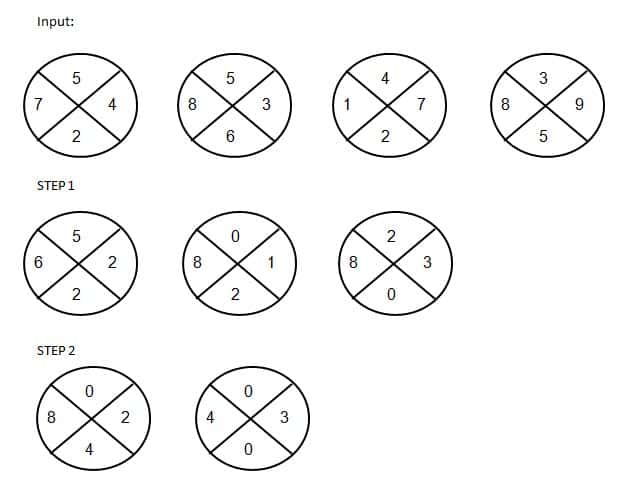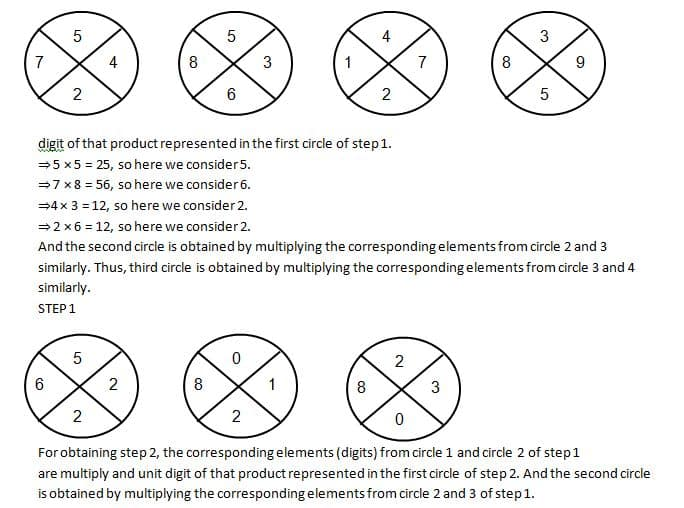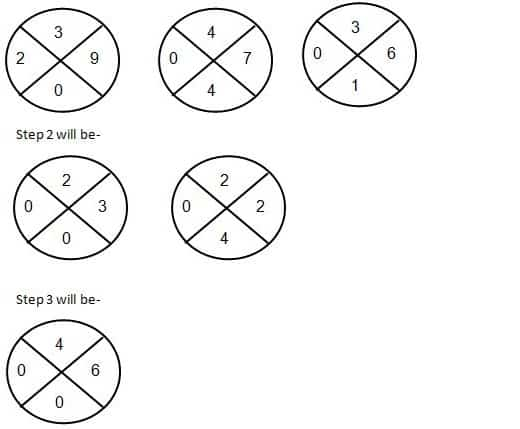# Reasoning Questions: Machine Input-Output Set 41

Hello Aspirants.

Welcome to Online Reasoning Section with explanation in AffairsCloud.com. Here we are creating Best question samples from Machine Input-output, which is common for all the IBPS, SBI , LIC, and other competitive exams. We have included questions that are repeatedly asked in bank exams !!!

(Direction 1 – 5): Study the given information carefully and answer the following questions.

A word arrangement machine when given an input of words, rearranges them following a particular rule in each step. The following is an illustration of input and steps rearrangement.
INPUT: Mobile Bag Plate Charger Mouse Box
STEP 1: Bag Mobile Plate Charger Mouse Box
STEP 2: Bag Box Mobile Plate Charger Mouse
STEP 3: Bag Box Charger Mobile Plate Mouse
STEP 4: Bag Box Charger Mobile Mouse Plate
This is the final arrangement and step 4 is the last step.
As per the rules followed in the given steps, answer the questions given below for the following input.
Input: Rent Dear House Bear Colony

1. What would be the last step of the following input?
1) Bear Colony Dear House Rent
2) Dear Bear House Colony Rent
3) Rent Dear House Bear Colony
4) Bear Colony House Rent Dear
5) Bear Colony Dear Rent House
Answer
Answer – 1) Bear Colony Dear House Rent
Solution:
Logic:
1) The rearrangement is taking place from left to right.
2) The rearrangement is taking place one word at a time.
In the following arrangement, words in each step are arranged in an increasing alphabetical order.
Input: Rent Dear House Bear Colony
Step 1: Bear Rent Dear House Colony
Step 2: Bear Colony Rent Dear House
Step 3: Bear Colony Dear Rent House
Step 4: Bear Colony Dear House Rent
Hence step 4 is the last step.
Hence, Bear Colony Dear House Rent is the last step of following input.
2. How many steps are there?
1) 3
2) 4
3) 5
4) 6
5) Either 3 or 5
Answer
Answer – 2) 4
Hence, there are 4 steps.
3. Which comes exactly between “Colony” and “House” in the final step?
1) Dear
2) Bear
3) Rent
4) Either Bear or Rent
5) None of these
Answer
Answer – 1) Dear
Hence, “Dear” comes exactly between “Colony” and “House” in the final step.
4. Which element is 3rd from the left in the final step?
1) Bear
2) Dear
3) Rent
4) House
5) Colony
Answer
Answer – 2) Dear
Hence, 3rd element from the left in the final step is “Dear”.
5. What would be the penultimate step of the given input?
1) Bear Colony Rent Dear House
2) Bear Rent Dear House Colony
3) Bear Colony Dear Rent House
4) Bear Colony Dear House Rent
5) None of the above
Answer
Answer – 3) Bear Colony Dear Rent House
Hence, “Bear Colony Dear Rent House” is the penultimate step of the given input.

(Directions 6 – 10): Study the following information to answer the given questions.
Given below an input-output arrangement, steps responsive mathematical operations on a set of numbers according to which you need to answer the following questions. The same operation is used in all the steps.1. If the numbers 3 and 6 are reversed in step 1, what is the difference between the old output and new output (you have to adding those 4 numbers of last step)?
1) Zero
2) 1
3) 2
4) 3
5) None of these
Answer
Answer – 2) 1
Solution:The result is = 6 + 3 = 9.
If the numbers 3 and 6 are reversed in step 1 then, Step 1 will be-If the numbers 3 and 6 are reversed in step 1 then, the result is = 6 + 4 = 10.
Hence, the difference is 10 – 9 = 1.

2. If all the numbers in step 2 are added with each other and subtract by the answer obtained by added the numbers of step 3, what’s the result?’
1) Zero
2) 1
3) 2
4) 3
5) None of these
Answer
Answer – 5) None of these
Solution:
The addition of numbers of step 2: 4 + 3 + 0 + 0 + 0 + 4 + 4 + 1 = 16
The addition of numbers of step 3: 0 + 0 + 6 + 3 = 9
Hence, the result is = 16 – 9 = 7.
3. How many elements in step 2 are multiples of 2?
1) Zero
2) 1
3) 2
4) 3
5) None of these
Answer
Answer – 3) 2
Hence, only two elements are there.
4. What is the resultant if all the numbers of step 3 multiply with each other?
1) 18
2) 6
3) 3
4) 0
5) 12
Answer
Answer – 4) 0
Hence, if all the numbers of step 3 multiply with each other resultant will be 0.
5. What is the sum of all the numbers of step 1?
1) 42
2) 40
3) 39
4) 35
5) None of these
Answer
Answer – 1) 42
AffairsCloud Recommends IBPSGuide Mock Test